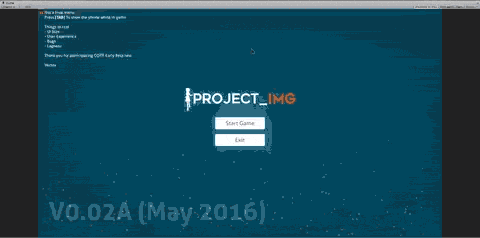# imagination XD

## realizing dream## [Unity UI 4.6] Parallax style menuSo yesterday I was trying to make parallax styled menu with Unity 4.6 UI, although it’s very simple to do.

1.2. Check your canvas setting, the render mode should be Screen Space Camera.
3.4. After that, add empty game object like Content. Content is like a group of everything that will be moved by script
5.6. Add this script on Canvas and set Canvas variable as your Content Rect transform.
7. also you need to adjust minMaxAxis, it’s an array, 0 usually -15, and 1 usually 15

Here’s the script (C#)

```using UnityEngine;
using UnityEngine.UI;
using System.Collections;

public class ParallaxMenu : MonoBehaviour {

public RectTransform canvas;
public float smoothTime = 0.3f;
public float minMaxXAxis = new float; //0 min, 1 max
public float minMaxYAxis = new float; //0 min, 1 max
public float mouseSensitivity = 50.0f;
private float xVelocity = 0.0f;
private float yVelocity = 0.0f;
private float x;
private float y;
private float xSmooth;
private float ySmooth;

void Awake(){
Cursor.lockState = CursorLockMode.None;
Cursor.visible = true;
}

void Update(){
x -= Input.GetAxis("Mouse X") * mouseSensitivity * 0.02f;
y -= Input.GetAxis("Mouse Y") * mouseSensitivity * .02f;

if(y > minMaxYAxis) y = minMaxYAxis;
if(y < minMaxYAxis) y = minMaxYAxis;
if(x > minMaxXAxis) x = minMaxXAxis;
if(x < minMaxXAxis) x = minMaxXAxis;

xSmooth = Mathf.SmoothDamp(xSmooth, x, ref xVelocity, smoothTime);
ySmooth = Mathf.SmoothDamp(ySmooth,y, ref yVelocity, smoothTime);

Quaternion parallax = new Quaternion();
parallax = Quaternion.Euler(ySmooth / 7,xSmooth/7,0);

canvas.transform.localPosition = new Vector3(xSmooth, ySmooth, 0f);
canvas.transform.localRotation = parallax;
}
}```